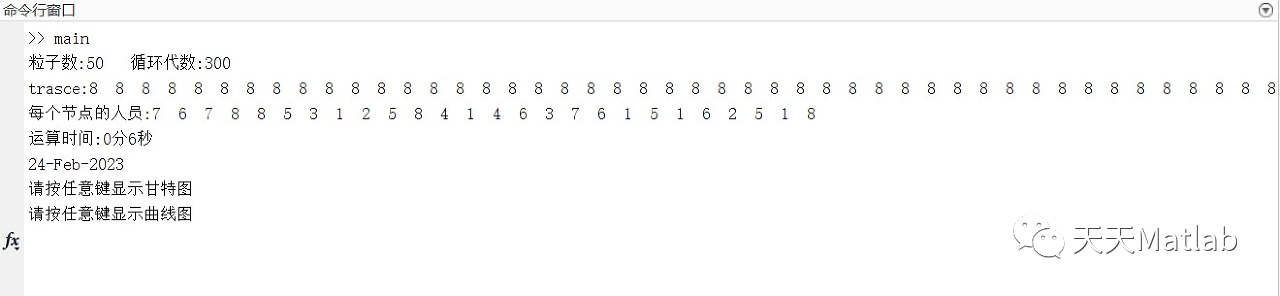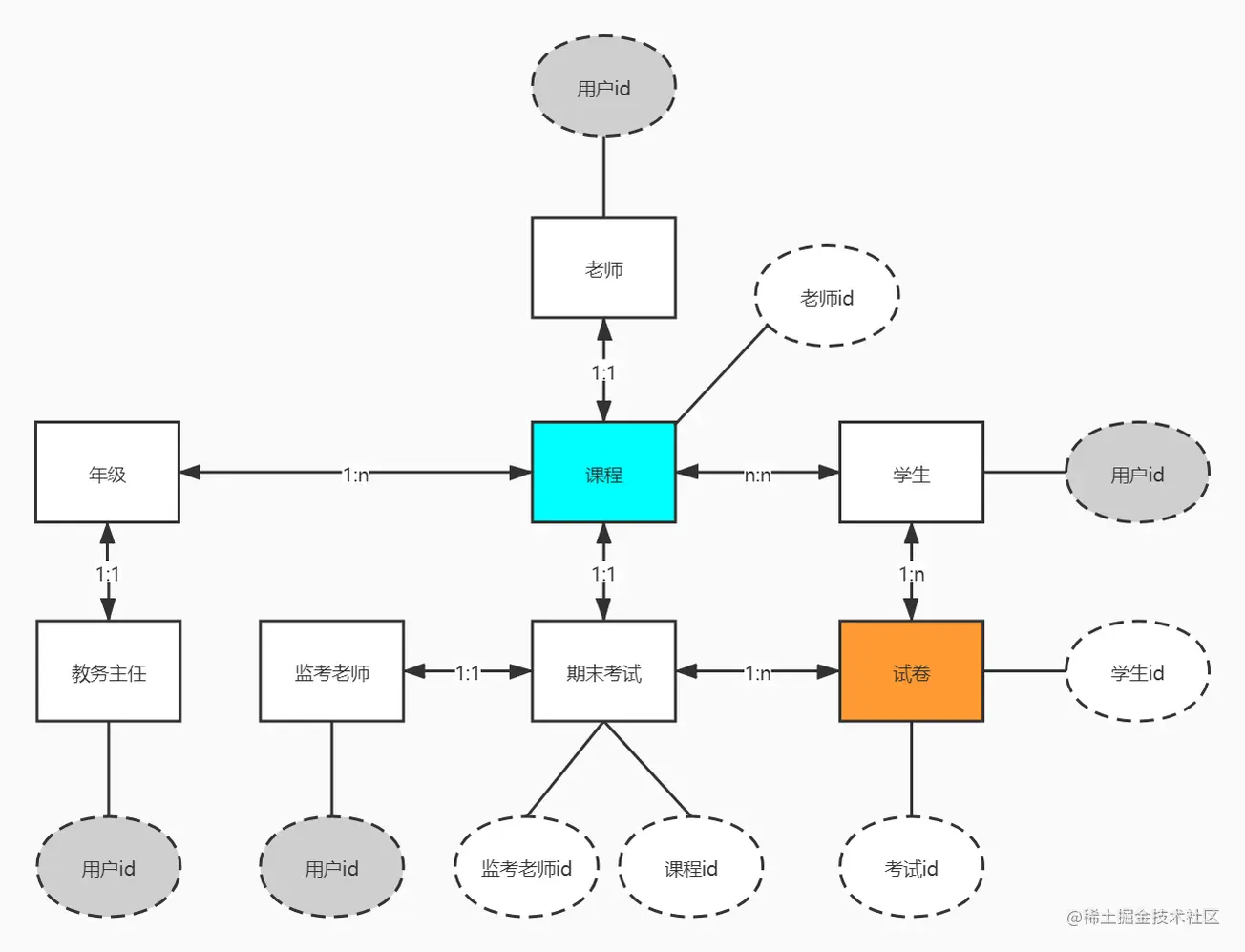# 《程序设计解题策略》——1.5　利用动态树维护森林的连通性

+关注继续查看

### 1.5　利用动态树维护森林的连通性

1.5.1　动态树的问题背景https://yqfile.alicdn.com/b1d50018c4f0ad81c62e5eda5d3a9c43a43b9956.png" >

1) MAKE TREE()：新建一棵仅有一个节点的树。
2) CUT(v)：删除v与它的父亲节点parent(v)的边，相当于将点v的子树分离了出来。
4) FIND_ROOT(v)：返回v所在的树的根节点。（1） Splay(p)

void Splay(node *p){　　　　　　　　// 把节点p旋到Splay的根部
while(p->f && (p->f->l==p || p->f->r==p)){
// 若p的父亲存在且p为其父的左右儿子之一，则调整
node *q=p->f,*y=q->f;// 记q为p的父亲，y为p的祖父
if (y && y->l==q){// 在q是y的左儿子的情况下，若p是q的左儿子，则q右旋后p右旋；
// 若p是q的右儿子，则p左旋右旋
if (q->l==p)zig(q),zig(p);
else zag(p),zig(p);
}else if (y && y->r==q){// 在q是y的左儿子的情况下,若p是q的右儿子，则q左旋后p左旋；
// 若p是q的左儿子，则p右旋左旋
if (q->r==p)zag(q),zag(p);
else zig(p),zag(p);
}else// 在q是树根的情况下，若p是q的左儿子，则p右旋；若p是q的
// 右儿子，则p左旋
{
if (q->l==p)zig(p);
else zag(p);
}
}
p->update();// 调整p子树的信息
}

<div style="text-align: center">
<img src=" https://yqfile.alicdn.com/8d47bf84772c21ec6c4cd106c7f4ffa66bbd9009.png" >
</div>
（2） Access(u)

Node access(Node u)
{
Node* v = EMPTY;　　　　　　　　　// 初始时v=EMPTY是为了清除u原来的孩子。因为不是每次Access
// 都需要把最后节点旋到Splay的根，所以不须最后Splay(v)
for (;u!=EMPTY;u=u->parent)// 向上追溯u至根的路径
{
splay(u);// 把节点u旋到Splay的根
u->child［1］ = v;// 把上一次的Splay的根节点v当作u的右孩子
update(v = u);// 将u记为v并调整其子树信息
}
return v;// 返回最后访问的节点v，即原树根
}

（3） MakeRoot(x)

inline void makeroot(Node* const x)
{
access(x)->rev ^= true;// 打通x到原根的路径，并打翻转标记（交换左右子树，也就是在原树中
// 交换上下关系）
splay(x);// 清除标记
}

（4） Find_Root(x)

Node Find_Root(Node x)// 构建一条一端是根、一端是x的Preferred_Path，然后不断往左走
// （往原树的根方向走），边走边清除标记，最终使得x变为所属Auxiliary Tree的最小节点
{
for (x=access(x); clear(x),x->child［0］!=EMPTY;x=x->child［0］);
return x;// 返回根节点
}

（5） Cut(x，y)

inline void cut(Node const x, Node const y)
{
makeroot(x);// 把x当作原树的根
access(y);// 构建一条一端是根、一端是y的Preferred_Path
splay(y);// 将y旋转至根
y->child［0］->parent=EMPTY;// 在y所属 Auxiliary Tree 中断开y与它的左子树的连接
y->child［0］ = EMPTY;
update(y);// 更新y所在子树的信息
}

（6） Link(x，y)

inline voidLink(Node const x, Node const y)
{
makeroot(x);// 把x当作原树的根
x->parent = y;// 修改 x所属的Auxiliary Tree的Path_Parent为y
access(x);// 构建一条一端是根、一端是x的Preferred_Path
}

（7） Query(x，y)

inline int query(Node x, Node y)
{
makeroot(x);// 把x当作原树的根
access(y), splay(y);// 构建一条一端是根、一端是y的Preferred_Path，将y旋转至根
return y->max;// 返回y子树的最大值
}

（8） Modify(x，y)

inline void modify(Node x, Node y, const int w)
{
makeroot(x);// 把x当作原树的根
access(y), splay(y);// 构建一条一端是根、一端是y的Preferred_ Path，将y旋转至根
_inc(y, w);// 同Splay中的清除标记
}

（9） splay_parent(x，y)

inline bool _splay_parent(Node x, Node (&y))
{ return (y=x->parent)!=EMPTY &&(y->child［0］==x ||y->child［1］==x); }

从上述9种操作的伪代码中可以看出，除Access操作之外的其他操作，其均摊时间复杂度至多为O(logN)，而每一个动态树操作都需要用到1次Access()，所以只用分析Access()的时间复杂度。

**1.5.4　应用动态树解题**

【1.5.1　洞穴勘测】
【问题描述】

<div style="text-align: center">
<img src="https://yqfile.alicdn.com/ee8b3cccc8c5dda050c16b18c0dba2e9b689ec44.png " >
</div>

10%的数据满足n≤1000，m≤20000
20%的数据满足n≤2000，m≤40000
30%的数据满足n≤3000，m≤60000
40%的数据满足n≤4000，m≤80000
50%的数据满足n≤5000，m≤100000
60%的数据满足n≤6000，m≤120000
70%的数据满足n≤7000，m≤140000
80%的数据满足n≤8000，m≤160000
90%的数据满足n≤9000，m≤180000
100%的数据满足n≤10000，m≤200000

保证所有Destroy指令将摧毁的是一条存在的通道。本题输入、输出规模比较大，建议C＼C++选手使用scanf和printf进行I＼O操作以免超时

“Destroy u v”命令需要将两个节点u、v间的边删除，即将一棵树切割为两棵树(对应cut操作)。我们首先要选出两个节点中深度较大的点。计算一个节点v深度的简单方法是先access(v)，然后splay(v)，v的左子树就是根到v这一条链上的节点。如果v不是根节点，v的左子树大小就是v的深度。设u的深度较浅。我们需要先access(v)。由于u一定是v的parent节点，因此我们splay(u)后直接切断两点的边，即断开u在它的所属Auxiliary Tree中与它的左子树的连接即可。
“Query u v”命令就是通过执行Find_Root(u)和Find_Root(v)，找出含u的Prefer Path在原树最上方的节点rb和含v的Prefer Path在原树最上方的节点rb。若ra==rb，则说明节点ra和rb在同一棵树中，u和v间有通道；否则u和v间无通道。计算find_root()其实很简单，只要access后找到splay中最小的点即可。

# include

const int MAXN = 10002;　　　　　　　　　　　// 节点数上限
struct Node// 节点的结构定义
{
bool rev;// 翻转标记
Node parent, child［2］;// 父指针和左右儿子指针
} _memory［MAXN］, *EMPTY=_memory;// 动态树

inline void clear(Node* const x)// 节点x翻转
{
if (x == EMPTY) return;// 若x节点空,则退出
if (x->rev)// 若x翻转，则左右儿子的翻转标志取反
{
x->child［0］->rev ^= true;x->child［1］->rev ^= true;
std::swap(x->child［0］, x->child［1］);// 交换x的左右儿子
x->rev = false;// 撤去x的翻转标志
}
}

void rotate(Node* const x, const int c)// 节点x按c方向旋转
{
Node* const y = x->parent;
y->child［c^1］ = x->child［c］;
if (x->child［c］ != EMPTY) x->child［c］->parent = y;
x->parent = y->parent;
if (y->parent->child［0］ == y) x->parent->child［0］ = x; else
if (y->parent->child［1］ == y) x->parent->child［1］ = x;
x->child［c］ = y;
y->parent = x;
}

inline bool _splay_parent(Node const x, Node (&y))
// 看x的父亲y是否为x所在splay的父亲
{ return (y = x->parent) != EMPTY && (y->child［0］ == x || y->child［1］ == x); }

void splay(Node* const x)// 把节点x旋到splay的根部
{
clear(x);// 节点x翻转
for (Node y, z; _splay_parent(x, y); )
if (_splay_parent(y, z))// 若y的父亲z为y所在splay的父亲
{
clear(z), clear(y), clear(x);// x、y和z翻转
const int c = y == z->child［0］;// 根据y在z的儿子方向，确定旋转方向
// 若x是y的c方向的儿子，则x先按c的相反方向旋转，
// 再按c方向旋转；若x是y的c的相反方向的儿子，则y和x先后按c方向旋转
if (x == y->child［c］) rotate(x, c^1), rotate(x, c);
else rotate(y, c), rotate(x, c);
}
Else// y的父亲z非y所在splay的父亲
{
clear(y), clear(x);// y和x翻转
rotate(x, x == y->child［0］);// 若x非y的左儿子，则x左旋；否则x右旋
}
}

Node access(Node u)// 将u到根的路径打通成为实边，且u的孩子节点的实边变
// 成虚边，使得这条Prefer Path一端是根，一端是u
{
Node* v = EMPTY;// 初始时v=EMPTY是为了翻转u原来的孩子。因为不是每
// 次access都需要把最后节点旋到splay的根，所以不须最后splay(v)
for (; u != EMPTY; u = u->parent)// 向上追溯u至根的路径
{
splay(u);// 把节点u旋到splay的根部
u->child［1］ = v;// 把上一次splay的根节点v当作u的右孩子
v = u;// 记下本次的splay根节点
}
return v;// 返回最后访问到的节点v，即原树根
}

inline Node getroot(Node x)// 计算x所属的Prefer Path在原树最上方的节点
{
for (x = access(x);clear(x), x->child［0］ != EMPTY; x = x->child［0］);
// 构建一条一端是根、一端是x的Prefer Path，然后不断往左走（往原树的根方向走）, 边走边清除标记，最终使
// 得x变为所属Auxiliary Tree的最小节点
return x;// 返回x所属的Prefer Path在原树最上方的节点
}

inline void makeroot(Node* const x)// 把x当作原树的根
{
access(x)->rev ^= true;// 打通到原根的路径，并翻转标记取反（交换左右子树，也就
// 是在原树中交换上下关系）
splay(x);// 将x旋转到根,即翻转
}

void link(Node const x, Node const y)// 把x和y所在的子树连接起来

{
makeroot(x);// 把x当作原树的根
x->parent=y;// 修改x所属的Auxiliary Tree的Path Parent为y
access(x);// 构建一条一端是根、一端是x的Prefer Path
}

void cut(Node const x, Node const y)// 以x为树根，把y到x的路径分离出来
{
makeroot(x);// 把x当作原树的根
access(y), splay(y);// 构建一条一端是根、一端是y的Prefer Path, 将y旋转至根
y->child［0］->parent=EMPTY;y->child［0］=EMPTY;
// 断开y在它的所属 Auxiliary Tree 中与它的左子树的连接
}

int n, m;// 洞穴数和指令数
int main()
{
scanf("%d%d", &n, &m);// 输入洞穴数和指令数
for (int i = 0; i <= n; ++i)// 动态树初始化为空
{
Node* const node = _memory+i;
node->child［0］ = node->child［1］ = node->parent = EMPTY;
}
for (int i = 0, x, y; i < m; ++i)// 处理每条命令
{
static char buf［10］;
scanf("＼n%s%d%d", buf, &x, &y);// 输入命令buf和参数x、y
Node ra, rb;
switch (buf［0］)
{
case 'Q':// 若询问命令
ra=getroot(_memory+x), rb=getroot(_memory+y);
// 计算含x的Prefer Path在原树的最上方节点ra和含y的Prefer Path在原树的最上方节点ra
printf(ra == rb && ra != EMPTY ? "Yes＼n" : "No＼n"); break;
// 若ra和rb在同一棵树，则洞穴x和洞穴y可互相连通；否则不连通
case 'D': cut(_memory+x, _memory+y); break;
// 若洞穴x和洞穴y之间的通道被毁，则以_memory［x］为树根，把_memory［y］到_memory［x］的路径分离出来
// 若洞穴x和洞穴y之间出现通道，则把_memory［x］和_memory［y］所在的子树连接起来
}
}
}

如果说［1.5.1 Cave洞穴勘测］是计算无权森林中树的合并、分离和询问节点间的连通关系，那么，我们再通过一个范例将问题拓展至有权森林中的路径计算。
【1.5.2　Tree】
【问题描述】

<div style="text-align: center">
<img src=" https://yqfile.alicdn.com/83c9c375cf7213bf137232785d72299fca86e246.png" >
</div>

<div style="text-align: center">
<img src=" https://yqfile.alicdn.com/5549a472088152ba2b45662bbf4af2811e20585d.png" >
</div>

1) 询问两点之间路径的边中边权最大的。
2) 把两点之间的路径的边权全部取反。
3) 修改某条边的边权。

# define INF 0x7fffffff// 定义无穷大

int N,q［MAXD］,first［MAXD］,e,next［MAXM］,v［MAXM］,w［MAXM］,dep［MAXD］;
// 节点数n，队列q［］，节点x在邻接表的首条边序号为first［x］，该边的端点v［e］，权w［e］，后继边next［e］；
// 节点x的度dep［x］
struct Edge// 边的结构定义
{
int x, y, z;// 权为z的边表(x,y)
}edge［MAXD］;// 边表
struct Splay// 伸展树节点的结构定义
{
int pre, ls, rs, neg, key, max, min;// 父指针、左右儿子指针、取反标志，边权、子树的最大值和最小值
bool root;// 当前节点是否为它所在的Splay tree的根标志
void update(); void pushdown(); void zig(int ); void zag(int ); void splay(int );
// 伸展树操作：调整子树的最大值和最小值update()，取反操作pushdown()、右旋操作zig(int )、左旋操作
// zag，通过一系列旋转将边调整至伸展树的根部splay(int )
void renew()// 构造单个节点的sply树
{
root = true; pre = ls = rs = 0; neg = 0;
}
}sp［MAXD］;// 伸展树sp［］

int Max(int x, int y)// 计算x和y的较大值
{
return x > y ? x : y;
}

int Min(int x, int y)// 计算x和y的较小值
{
return x < y ? x : y;
}

void Splay::update()// 伸展树操作：调整子树的最大值和最小值
{
max = Max(Max(sp［ls］.max, sp［rs］.max), key);
min = Min(Min(sp［ls］.min, sp［rs］.min), key);
}

void makeneg(int cur)// 节点cur取反
{
if(cur!=0)// 节点cur的取反标志取反。该边的关键字取负，max域取
// min域的负值，min域取max域的负值
{
sp［cur］.neg ^= 1, sp［cur］.key = -sp［cur］.key;
int t = sp［cur］.max;sp［cur］.max = -sp［cur］.min, sp［cur］.min = -t;
}
}

void Splay::pushdown()// 伸展树操作：下传取反的懒惰标志
{
if(neg)// 若取反的懒惰标志存在，则下传给左右儿子，撤去其懒惰标志
{
makeneg(ls), makeneg(rs); neg = 0;
}
}

void Splay::zig(int x)// 伸展树操作：节点x右旋操作
{
int y = rs, fa = pre;
pushdown(), sp［y］.pushdown();
rs = sp［y］.ls, sp［rs］.pre = x;
sp［y］.ls = x, pre = y; sp［y］.pre = fa;
if(root) root = false, sp［y］.root = true;
else sp［fa］.rs == x ? sp［fa］.rs = y : sp［fa］.ls = y;
update();
}
void Splay::zag(int x)// 伸展树操作：节点x左旋操作
{
int y = ls, fa = pre;
pushdown(), sp［y］.pushdown();
ls = sp［y］.rs, sp［ls］.pre = x;
sp［y］.rs = x, pre = y; sp［y］.pre = fa;
if(root) root = false, sp［y］.root = true;
else sp［fa］.rs == x ? sp［fa］.rs = y : sp［fa］.ls = y;
update();
}
void Splay::splay(int x)// 保持伸展树有序性的前提下，通过一系列旋转将伸展树中
// 的元素x调整至树的根部，维护x子树的最大值和最小值
{
int y, z;
for(pushdown(); !root;)
{
y = pre;
if(sp［y］.root) sp［y］.rs == x ? sp［y］.zig(y) : sp［y］.zag(y);
else
{
z = sp［y］.pre;
if(sp［z］.rs == y)
{
if(sp［y］.rs == x) sp［z］.zig(z), sp［y］.zig(y);
else sp［y］.zag(y), sp［z］.zig(z);
}
else
{
if(sp［y］.ls == x)
sp［z］.zag(z), sp［y］.zag(y);
else
sp［y］.zig(y), sp［z］.zag(z);
}
}
}
update();
}

void add(int x, int y, int z)// 将权为z的边(x,y)加入邻接表
{
v［e］ = y, w［e］ = z;
next［e］ = first［x］, first［x］ = e ++;
}

void prepare()// 计算树中每个节点的父指针、层次、key、max和min域初始化
{
int i, j, x, rear = 0;// 队尾指针初始化
sp［0］.max = -INF, sp［0］.min = INF;
q［rear ++］ = 1;// 节点1入队
sp［1］.renew(), dep［1］ = 1;// 为节点1申请内存，层次为1
for(i = 0; i < rear; i ++)// 队列中的节点依次出队，直至队列空
{
x = q［i］;
for(j = first［x］; j != -1; j = next［j］)
// 搜索x的每条出边，设定儿子节点的父指针、层次、key、max和min域设为边权，儿子入队
if(v［j］ != sp［x］.pre)
{
sp［v［j］］.renew(), sp［v［j］］.pre = x, dep［v［j］］ = dep［x］+1;
sp［v［j］］.key = sp［v［j］］.max = sp［v［j］］.min=w［j］;
q［rear ++］ = v［j］;
}
}
}

void Swap(int &x, int &y)// 交换x和y
{
int t;
t = x, x = y, y = t;
}

void init()// 输入信息，构造相邻矩阵
{
int i;
memset(first, -1, sizeof(first));
e = 0;
scanf("%d", &N);// 输入节点数
for(i = 1; i < N; i ++)// 输入每n-1条边信息，构建邻接表
{
scanf("%d%d%d", &edge［i］.x, &edge［i］.y, &edge［i］.z);
}
prepare();// 计算树中每个节点的父指针、层次、key、max和min域初始化
for(i = 1; i < N; i ++)// 每条边x端节点的深度大
if(dep［edge［i］.x］>dep［edge［i］.y］)Swap(edge［i］.x, edge［i］.y);
}

void access(int x)// 从节点x到根上的所有点按深度用一棵splay维护，左边
// 比根深度小，右边比根深度大
{
int fx;
for(fx=x,x=0;fx!=0;x=fx,fx=sp［x］.pre)
// 依次枚举x至根路径上的每个节点fx
{
sp［fx］.splay(fx);sp［sp［fx］.rs］.root=true;
// 将fx旋转至根，fx的右儿子设旋转标志
sp［fx］.rs=x,sp［x］.root=false;sp［fx］.update();
// fx的右儿子设为x，x不再作为splay的根，维护fx子树的最大值和最小值
}
}

void Change(int x, int y)// 将节点x的出边权值改为y
{
sp［x］.splay(x); sp［x］.key = y; sp［x］.update();
// 将节点x调整到树的根部，将出边权改为y，维护其子树的最大值和最小值
}

void Negate(int x, int y)// 消除x到y路上每条边的权值
{
int fy;
access(x);// 从节点x到根上的所有点按深度用一棵splay维护，左边
// 比根深度小，右边比根深度大
for(fy=y, y=0;fy!=0;y=fy,fy=sp［y］.pre)
// 依次枚举y至根路径上的每个节点fy
{
sp［fy］.splay(fy);// 将fy旋转至根
if(sp［fy］.pre == 0) makeneg(sp［fy］.rs), makeneg(y);
// 若fy为根，其右儿子取反，后继节点y取反
sp［sp［fy］.rs］.root=true;sp［fy］.rs=y,sp［y］.root=false;
// 设fy的右儿子为splay的根标志，fy的右儿子更新为y，取消y的根标志
sp［fy］.update();// 维护fy子树的最大值和最小值
}
}

void Query(int x, int y)// 计算和输出x到y路上的最大权值
{
int fy;
access(x);// 从节点x到根上的所有点按深度用一棵splay维护，左边
// 比根深度小，右边比根深度大
for(fy = y, y = 0; fy != 0; y = fy, fy = sp［y］.pre)
// 依次枚举y至根路径上的每个节点fy（其后继节点为y）
{
sp［fy］.splay(fy);// 将fy旋转至根
if(sp［fy］.pre == 0)// 若fy为根，则x到y路上的最大权值为max{fy右子树的
// 最大值,y子树的最大值}
printf("%d＼n", Max(sp［sp［fy］.rs］.max, sp［y］.max));
sp［sp［fy］.rs］.root = true; sp［fy］.rs = y, sp［y］.root = false;
// 设splay的根为fy的右儿子，y调整为fy的右儿子，取消y的根标志
sp［fy］.update();// 维护fy子树的最大值和最小值
}
}

void solve()// 依次处理当前测试用例的每条命令
{
int x, y;// 命令参数
char op［10］;// 命令字
for(;;)
{
scanf("%s", op);// 输入命令字
if(op［0］ == 'D') break;// 若为结束命令，则退出
scanf("%d%d", &x, &y);// 输入命令参数
if(op［0］ == 'C') Change(edge［x］.y, y);
// 将第x条边的权值改为y
else if(op［0］ == 'N') Negate(x, y);
// 消除x到y路上每条边的权值
else Query(x, y);// 计算和输出x到y路上的最大权值
}
}

int main()
{
int t;
scanf("%d", &t);// 输入测试用例数
while(t --)// 依次处理每个测试用例
{
init();// 输入信息
solve();// 依次处理当前测试用例的每条命令
}
return 0;
}【优化指派】基于粒子群算法求解指派优化工作质量问题附matlab代码
【优化指派】基于粒子群算法求解指派优化工作质量问题附matlab代码
15 0206 01791. 找出星型图的中心节点 : 简单模拟题（进阶到欧拉回路问题）
1791. 找出星型图的中心节点 : 简单模拟题（进阶到欧拉回路问题）
42 0【回溯算法】经典题：求目标和的组合方案 ...｜刷题打卡
【回溯算法】经典题：求目标和的组合方案 ...｜刷题打卡
105 0【回溯算法】求目标和的组合方案（升级篇） ... ｜刷题打卡
【回溯算法】求目标和的组合方案（升级篇） ... ｜刷题打卡
56 04262 01360 01153 0RIPv1和v2综合实验试题的(答案和配置过程)
1268 0

+ 订阅